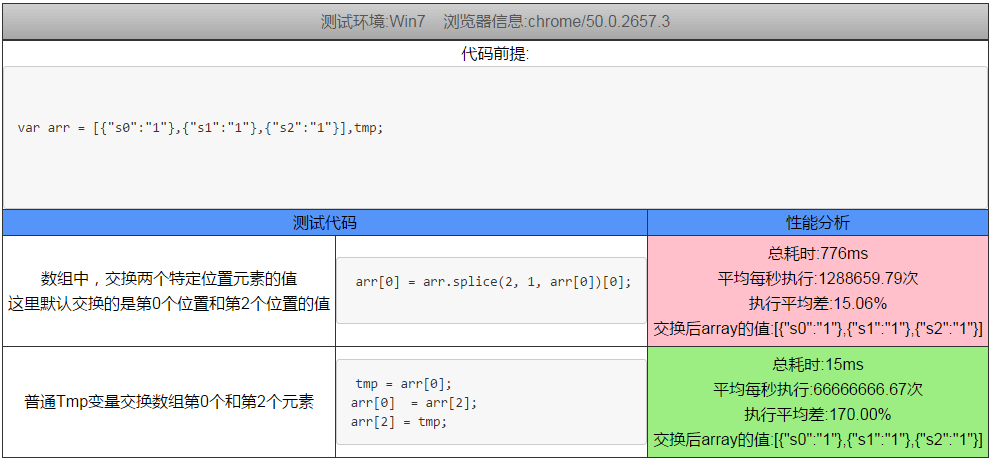# JS几种变量交换方式以及性能分析对比

| 分类 blog  | | 浏览

## 前言

“两个变量之间的值得交换”，这是一个经典的话题，现在也有了很多的成熟解决方案，本文主要是列举几种常用的方案，进行大量计算并分析对比。

## 起由

``````arr = [item0,item1,...,itemN];
//最初使用这段代码来交换第0个和第K(k<N)个元素
arr = arr.splice(k, 1, arr);
``````## JS变量交换的几种方式

### 第一种:普通临时变量交换方式

``````tmp = a;
a = b;
b = tmp;
``````

(在JS中,这种方式效率确实很高，而且就算是其它语言中，只要tmp变量提前创建，性能也不会很低，反而是一些杂技派和少数派性能方面很低)

### 第二种:利用一个新的对象来进行数据交换

``````a = {a : b, b : a};
b = a.b
;a = a.a;
``````

### 第三种:利用一个新的数组来进行数据交换

``````a = [b, b=a];
``````

### 第四种:利用数组交换变量(需EJS支持)

```````[a, b] = [b, a];
``````

### 第五种:利用try catch交换

``````a=(function(){;
try{return b}
finally{b=a}}
)();
``````

### 第六种:异或操作交换变量第一种方式

``````a = (b = (a ^= b) ^ b) ^ a;
``````

### 第七种:异或操作交换变量第二种方式

``````a ^=b;
b ^=a;
a ^=b;
``````

### 第八种:数字之间的加减运算来实现，第一种加减方式

``````a = a + b;
b = a - b;
a = a - b;
``````

### 第九种:数字之间的加减运算来实现，第一种加减方式

``````a = b -a +(b = a);
``````

### 第十种:利用eval计算

``````eval("a="+b+";b="+a);
``````

### 第十一种:数组中，利用splice交换两个元素的位置

``````arr = arr.splice(2, 1, arr);
``````

## 各种交换方式的性能对比

### 性能对比截图

#### 分析结果2可以看出,eval最慢,splice性能较低，tmp变量交换很稳定

JS几种变量交换方式分析比较

## 原文链接

JS几种变量交换方式以及性能分析对比

## 参考

Exchange Variables Gracefully